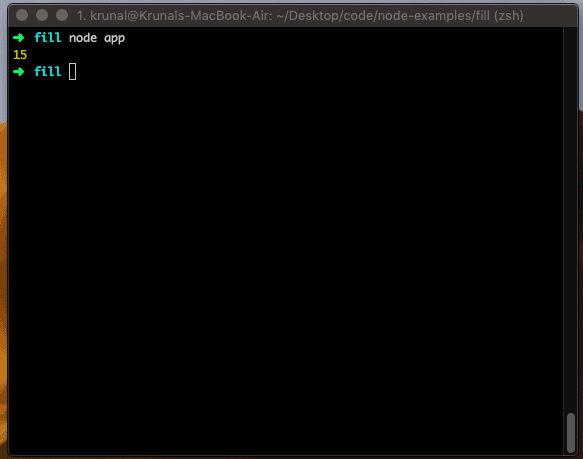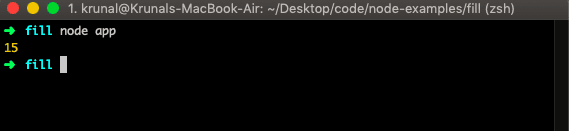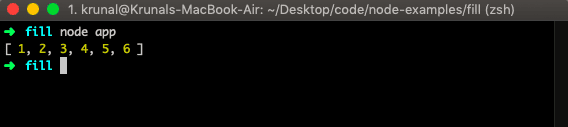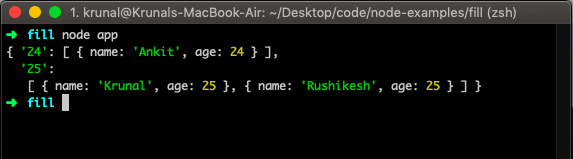# JavaScript reduce: The Complete Guide

JavaScript reduce() method does not execute a function for array items without values. Instead, the reduce() method reduces the array to a single value. The reduce() method provides a function for each array value (left to right). The function’s return value is stored in an accumulator (result/total).

## JavaScript reduce

The array reduce() is a built-in JavaScript function that executes the reducer function on each element, resulting in a single output value. The reduce() method reduces an array to a single value. The reduce() method executes the provided function for each array value (from left to right). The return value of the function is stored in the total.

### Syntax

The syntax of the reduce() method is the following.

`array.reduce(function(total, currentValue, currentIndex, arr), initialValue)`

### Parameters

The total argument is required. It is the initial value or the previously returned value of the function.

CurrentValue is the required argument. It is the value of the current item in an array.

The currentIndex is an optional argument. It is the index of the current element.

The arr is an optional argument. It is the array object the current element belongs to.

The initialValue is an optional argument. It is a value to be passed to the function as the initial value.

The first time the callback is called total, and currentValue can be one of two values. For example, if an initialValue is provided in the call to the reduce() method, the total will be equal to the initialValue, and the currentValue will equal the first value in the array.

If no initialValue is provided, then the total will be equal to the first item in the array, and currentValue will be equal to the second.

### Example

Let us take an easy example of the array reduce() method.

Create a file called app.js and write the following code inside the app.js file. We use Node.js to run the file and display the output on the terminal.

```// app.js

let arr = [1, 2, 3, 4, 5];
let sum = 0;
for(var i = 0; i < arr.length; i++) {
sum += arr[i];
}
console.log(sum);```

So, in the above example, we have used the for loop to sum all the array’s items. We can do the same thing using the JavaScript reduce() method.

```// app.js

let arr = [1, 2, 3, 4, 5];

let sum = arr.reduce((x, y) => x + y);

console.log(sum);```

Now run the file, and the output still will be 15.

#### OutputIf the array is empty and has no initialValue, then TypeError will be thrown. If an array has only one item (regardless of position) and no initialValue is provided, or if initialValue is provided, but an array is empty. The single value will be returned without calling the callback function.

So, it performs like this.

```[1, 2, 3, 4, 5].reduce(function(total, currentValue, currentIndex, array) {
});```

The value returned by the reduce() would be that of the last callback invocation (15).

## Sum of values in an object array

Write the following code.

```// app.js

let initialValue = 0;
let objArray = [
{x: 1},
{x: 2},
{x: 3},
{x: 4},
{x: 5},
]
let sum = objArray.reduce(function (total, currentValue) {
}, initialValue);

console.log(sum);```

So, in the above example, we have defined the initialValue to 0 and then passed the object values to the next, and then it will sum up all the values of all the objects and returns the output.

#### Output## Flatten an array of arrays in Javascript

To flatten the array of arrays in Javascript, we can use the Javascript array reduce() method. The reduce() method flattened the array of arrays into one with the individual items.

```// app.js

let flattened = [[1, 2], [3, 4], [5, 6]]
.reduce((total, currentValue) => total.concat(currentValue), []);

console.log(flattened);```

#### OutputIn the above example, we have defined a two-dimensional array and then used the reduce() method to flatten that array into a one-dimensional array using array.concat() method.

The concat method takes the value one by one and puts them into the empty array; hence all the individual values have been put into an empty array, and finally, we get the flattened array.

## Grouping JavaScript objects by a property

In this example, we will group the object based on their age numbers. It is a problematic example, but it will become easy for you after you understand it.

```// app.js

const people = [
{ name: 'Krunal', age: 25 },
{ name: 'Rushikesh', age: 25 },
{ name: 'Ankit', age: 24 }
];

const groupBy = (objectArray, property) => {
return objectArray.reduce(function (total, obj) {
let key = obj[property];
if (!total[key]) {
total[key] = [];
}
total[key].push(obj);
}, {});
}

let groupedPeople = groupBy(people, 'age');

console.log(groupedPeople);```

The above example will form an array of objects whose key is the age number, and its properties are objects with that same age number. So we are grouping the people based on their ages.

#### OutputYou can perform complex operations using the reduce() methodOne of the most prominent examples is Redux. It uses the same pattern.

That’s it for this tutorial.

Javascript array push()

Javascript array filter()

Javascript array map()

Javascript array forEach()

Javascript array unshift()

### 2 thoughts on “JavaScript reduce: The Complete Guide”

1.thanks for the post

2.